### Home > CALC > Chapter Ch3 > Lesson 3.1.1 > Problem3-16

3-16.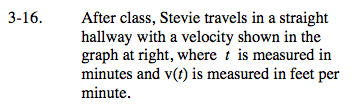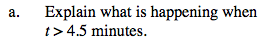Notice that the velocity is negative when t > 4.5.

When velocity is negative, Stevie moves back towards his starting position.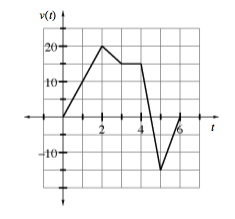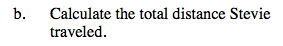Total distance is the area under a speed graph. Speed is the absolute value of velocity.

A speed graph is the absolute value of a velocity graph...
Area under a speed graph = total distance.
Area under a velocity graph = displacement.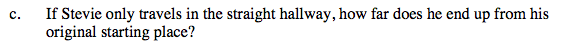Distance from starting point is known as 'displacement.'
Displacement is the area under a velocity graph.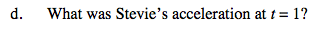Acceleration is slope on a velocity graph.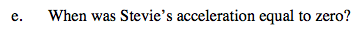See hint in part (d).